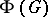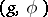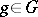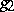# Holomorph of a group

(diff) ← Older revision | Latest revision (diff) | Newer revision → (diff)

A concept in group theory which arose in connection with the following problem. Is it possible to include any given groupas a normal subgroup in some other group so that all the automorphisms ofare restrictions of inner automorphisms of this large group? To solve a problem of this kind, a new groupis constructed usingand its automorphism group. The elements ofare pairswhere,, and composition of pairs is defined by the formulawhereis the image ofunder. The group(or a group isomorphic to it) is called the holomorph of. The set of pairs of the form, whereis the identity element of, constitutes a subgroup that is isomorphic to the original group. In a similar manner, the pairs of the form, whereis the identity element of, constitute a subgroup isomorphic to the group. The formulashows thatis in fact a solution of the problem posed above.